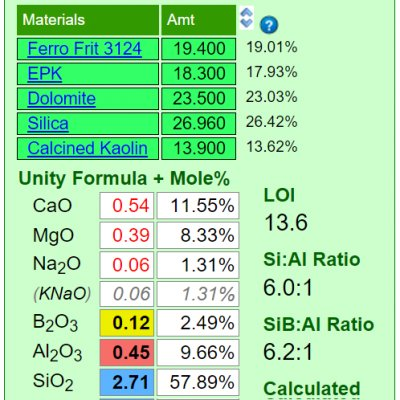Monthly Tech-Tip from Tony Hansen SignUp No tracking! No ads!

# Unity Formula

The chemistry of ceramic glazes are normally expressed as formulas. A unity formula has been retotaled to make the numbers of flux oxides total one.

## Details

A "unity formula" is just an oxide formula that has been retotaled so that the RO group of oxides sums to 1. This is also called a Seger formula. It is a standard that provides one basis for comparing glazes. The three-column format of expressing a formula was first used by Hermann Seger. Here is how we would recalculate a raw formula to a flux unity:

```           Raw              Unity
Oxides     Formula          Formula
-----------------------------------
K2O         0.6  /  2.2  =  0.27
CaO         1.3  /  2.2  =  0.59
MgO         0.2  /  2.2  =  0.09
ZnO         0.1  /  2.2  =  0.05
-----           ----
Flux Total  2.2              1.0
Al2O3       0.9  /  2.2  =  0.41
SiO2        9.0  /  2.2  =  4.09
-----------------------------------```

The Seger method of rationalizing the chemistry of glazes does not work as well at lower temperatures because some oxides that are powerful fluxes at high temperatures are refractory or inactive in low fire. Oxides have a much more individual presence or depend on interactions (at each temperature range) than the Seger method tends to recognize. Also, their contributions to particular properties often are not linear according to concentration. For example, boron is both a glass and a flux and the logic for its employment at various temperature ranges differs. It does not 'plug into' a Seger formula well. Many people prefer to use Mole% when rationalizing how a formula relates to the fired product. However, all of this being said, there is great value in a method of presentation that clearly shows the relationship between Al2O3, SiO2 and everything else.

Some argue that the unity formula method of presenting glazes is not precise enough for glaze chemistry calculations. However, the greatest source of imprecision by far is the accuracy of the analyses of the materials being used. Any method that calculates an exact match for an existing chemistry will also produce an exact match in unity formulas. Unity formulas work better in targeting when doing batch to chemistry calculations because additions or changes in percentage of a material do not always change every number in the formula. However it must be remembered that glaze chemistry is not about precise mathematics, it is comparative and best viewed in context and as a tool to help when comparing one thing with another.

Digitalfire desktop Insight required that users specify whether to unify recipes to RO or R2O3. However Insight-live automatically sets this (the group having the greater total wins the unity setting). Users can click the 'Auto Unity' heading and it will cycle between various settings, eventually coming back to auto-unity. Recipes remember their settings.

## Related Information

### A Limitation of the Seger Unity Formula

Tap picture for full size and resolutionMineral sources of oxides impose their own melting patterns and when one is substituted for another to supply an oxide a different system with its own relative chemistry is entered. An extreme example of this would be to source Al2O3 to a glaze using calcined alumina instead of kaolin. Although the formula may be exactly the same, the fired result would be completely different because very little of the alumina would dissolve into the glaze melt. At the opposite extreme, a different frit could be used to supply a set of oxides (while maintaining the overall chemistry of the glaze) and the fired result would be much more chemically predictable. Why? Because the readily and release their oxides the the melt.

 By Tony Hansen